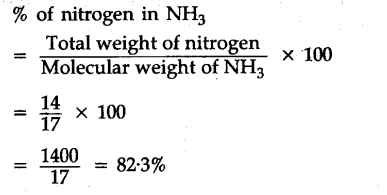# Calculate the percentage of nitrogen in N\${{H}_{3}}\$

Calculate the percentage of nitrogen in N\${{H}_{3}}\$
(Atomic mass of N =,14,-H = 1 amu)